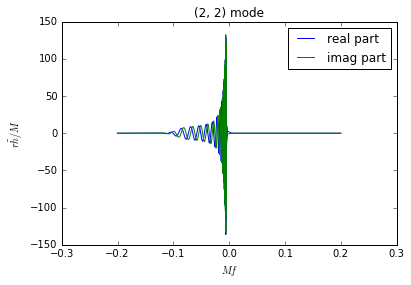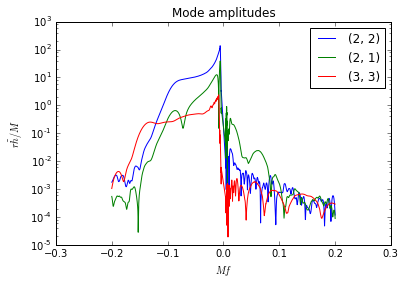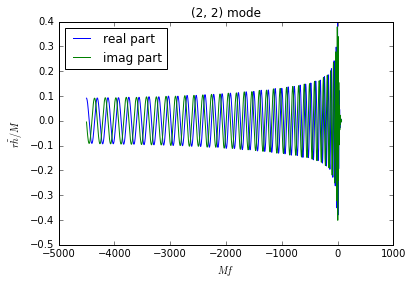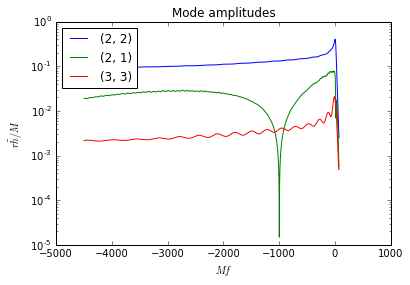In :
import gwsurrogate as gws # import gwsurrogate package
import gwtools  # import gwtools (a collection of useful python functions included with gwsurrogate)

%matplotlib inline
import numpy as np, matplotlib.pyplot as plt


Load the frequency domain surrogate:

In :
# Surrogate data located at http://www.black-holes.org/surrogates/
sur = gws.EvaluateSurrogate('NRSur4d2s_FDROM_grid12.h5', use_orbital_plane_symmetry=False)

loading surrogate mode... l2_m-1

>>> Warning: Guessing quadrature weights to be identical with 0.000200
Cannot load greedy points...OK
Cannot load eim points...OK
Special case: using fast tensor spline for real and imaginary parts instead of amp/phase

>>> Warning: Guessing quadrature weights to be identical with 0.000200
Cannot load greedy points...OK
Cannot load eim points...OK
Special case: using fast tensor spline for real and imaginary parts instead of amp/phase

>>> Warning: Guessing quadrature weights to be identical with 0.000200
Cannot load greedy points...OK
Cannot load eim points...OK
Special case: using fast tensor spline for real and imaginary parts instead of amp/phase

>>> Warning: Guessing quadrature weights to be identical with 0.000200
Cannot load greedy points...OK
Cannot load eim points...OK
Special case: using fast tensor spline for real and imaginary parts instead of amp/phase

>>> Warning: Guessing quadrature weights to be identical with 0.000200
Cannot load greedy points...OK
Cannot load eim points...OK
Special case: using fast tensor spline for real and imaginary parts instead of amp/phase

>>> Warning: Guessing quadrature weights to be identical with 0.000200
Cannot load greedy points...OK
Cannot load eim points...OK
Special case: using fast tensor spline for real and imaginary parts instead of amp/phase

>>> Warning: Guessing quadrature weights to be identical with 0.000200
Cannot load greedy points...OK
Cannot load eim points...OK
Special case: using fast tensor spline for real and imaginary parts instead of amp/phase

>>> Warning: Guessing quadrature weights to be identical with 0.000200
Cannot load greedy points...OK
Cannot load eim points...OK
Special case: using fast tensor spline for real and imaginary parts instead of amp/phase

>>> Warning: Guessing quadrature weights to be identical with 0.000200
Cannot load greedy points...OK
Cannot load eim points...OK
Special case: using fast tensor spline for real and imaginary parts instead of amp/phase

>>> Warning: Guessing quadrature weights to be identical with 0.000200
Cannot load greedy points...OK
Cannot load eim points...OK
Special case: using fast tensor spline for real and imaginary parts instead of amp/phase

>>> Warning: Guessing quadrature weights to be identical with 0.000200
Cannot load greedy points...OK
Cannot load eim points...OK
Special case: using fast tensor spline for real and imaginary parts instead of amp/phase

>>> Warning: Guessing quadrature weights to be identical with 0.000200
Cannot load greedy points...OK
Cannot load eim points...OK
Special case: using fast tensor spline for real and imaginary parts instead of amp/phase


Evaluate a waveform:

In :
q = 1.2
chiA_mag = 0.6
chiA_polar_angle = np.pi / 3 # \theta_\chi
chiA_azimuthal_angle = 0 # \phi_\chi
chiB_z = -0.3

x = np.array([q, chiA_mag, chiA_polar_angle, chiA_azimuthal_angle, chiB_z])

modes, freqs, h_real, h_imag = sur(x, fake_neg_modes=False, mode_sum=False)
hf = h_real + 1.j*h_imag

# Plot the (2, 2) mode
plt.plot(freqs, np.real(hf[:, 4]), label='real part')
plt.plot(freqs, np.imag(hf[:, 4]), label='imag part')
plt.title("(2, 2) mode")
plt.xlabel("$Mf$")
plt.ylabel("$r\\tilde{h}/M$")
plt.legend()
plt.show()

# Plot the amplitudes of various modes
plt.semilogy(freqs, abs(hf[:, 4]), label='(2, 2)')
plt.semilogy(freqs, abs(hf[:, 3]), label='(2, 1)')
plt.semilogy(freqs, abs(hf[:, 11]), label='(3, 3)')
plt.title("Mode amplitudes")
plt.xlabel("$Mf$")
plt.ylabel("$r\\tilde{h}/M$")
plt.legend()
plt.show()We also have a fast time-domain tensor spline ROM built in a similar way as NRSur4d2s_FDROM, but without the FFT step. We call this model NRSur4d2s_TDROM. It's loaded and evaluated in the same way:

In :
# Surrogate data located at http://www.black-holes.org/surrogates/
sur = gws.EvaluateSurrogate('NRSur4d2s_TDROM_grid12.h5', use_orbital_plane_symmetry=False)

loading surrogate mode... l2_m-1

>>> Warning: Guessing quadrature weights to be identical with 3.958740
Cannot load greedy points...OK
Cannot load eim points...OK
Special case: using fast tensor spline for real and imaginary parts instead of amp/phase

>>> Warning: Guessing quadrature weights to be identical with 3.958740
Cannot load greedy points...OK
Cannot load eim points...OK
Special case: using fast tensor spline for real and imaginary parts instead of amp/phase

>>> Warning: Guessing quadrature weights to be identical with 3.958740
Cannot load greedy points...OK
Cannot load eim points...OK
Special case: using fast tensor spline for real and imaginary parts instead of amp/phase

>>> Warning: Guessing quadrature weights to be identical with 3.958740
Cannot load greedy points...OK
Cannot load eim points...OK
Special case: using fast tensor spline for real and imaginary parts instead of amp/phase

>>> Warning: Guessing quadrature weights to be identical with 3.958740
Cannot load greedy points...OK
Cannot load eim points...OK
Special case: using fast tensor spline for real and imaginary parts instead of amp/phase

>>> Warning: Guessing quadrature weights to be identical with 3.958740
Cannot load greedy points...OK
Cannot load eim points...OK
Special case: using fast tensor spline for real and imaginary parts instead of amp/phase

>>> Warning: Guessing quadrature weights to be identical with 3.958740
Cannot load greedy points...OK
Cannot load eim points...OK
Special case: using fast tensor spline for real and imaginary parts instead of amp/phase

>>> Warning: Guessing quadrature weights to be identical with 3.958740
Cannot load greedy points...OK
Cannot load eim points...OK
Special case: using fast tensor spline for real and imaginary parts instead of amp/phase

>>> Warning: Guessing quadrature weights to be identical with 3.958740
Cannot load greedy points...OK
Cannot load eim points...OK
Special case: using fast tensor spline for real and imaginary parts instead of amp/phase

>>> Warning: Guessing quadrature weights to be identical with 3.958740
Cannot load greedy points...OK
Cannot load eim points...OK
Special case: using fast tensor spline for real and imaginary parts instead of amp/phase

>>> Warning: Guessing quadrature weights to be identical with 3.958740
Cannot load greedy points...OK
Cannot load eim points...OK
Special case: using fast tensor spline for real and imaginary parts instead of amp/phase

>>> Warning: Guessing quadrature weights to be identical with 3.958740
Cannot load greedy points...OK
Cannot load eim points...OK
Special case: using fast tensor spline for real and imaginary parts instead of amp/phase

In :
modes, times, h_real, h_imag = sur(x, fake_neg_modes=False, mode_sum=False)
hf = h_real + 1.j*h_imag

# Plot the (2, 2) mode
plt.plot(times, np.real(hf[:, 4]), label='real part')
plt.plot(times, np.imag(hf[:, 4]), label='imag part')
plt.title("(2, 2) mode")
plt.xlabel("$Mf$")
plt.ylabel("$r\\tilde{h}/M$")
plt.legend(loc='upper left')
plt.show()

# Plot the amplitudes of various modes
plt.semilogy(times, abs(hf[:, 4]), label='(2, 2)')
plt.semilogy(times, abs(hf[:, 3]), label='(2, 1)')
plt.semilogy(times, abs(hf[:, 11]), label='(3, 3)')
plt.title("Mode amplitudes")
plt.xlabel("$Mf$")
plt.ylabel("$r\\tilde{h}/M$")
plt.legend(loc='upper left')
plt.show()In [ ]: